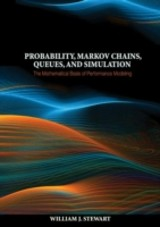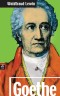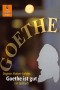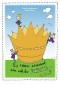# Details## Probability, Markov Chains, Queues, and Simulation

The Mathematical Basis of Performance Modeling

 147,00 € Verlag: Princeton University Press Format: EPUB, PDF Veröffentl.: 06.07.2009 ISBN/EAN: 9781400832811 Sprache: englisch Anzahl Seiten: 776

DRM-geschütztes eBook, Sie benötigen z.B. Adobe Digital Editions und eine Adobe ID zum Lesen.

## Beschreibungen

#### Titelkurzbeschreibung

Probability, Markov Chains, Queues, and Simulation provides a modern and authoritative treatment of the mathematical processes that underlie performance modeling. The detailed explanations of mathematical derivations and numerous illustrative examples make this textbook readily accessible to graduate and advanced undergraduate students taking courses in which stochastic processes play a fundamental role. The textbook is relevant to a wide variety of fields, including computer science, engineering, operations research, statistics, and mathematics.The textbook looks at the fundamentals of probability theory, from the basic concepts of set-based probability, through probability distributions, to bounds, limit theorems, and the laws of large numbers. Discrete and continuous-time Markov chains are analyzed from a theoretical and computational point of view. Topics include the Chapman-Kolmogorov equations; irreducibility; the potential, fundamental, and reachability matrices; random walk problems; reversibility; renewal processes; and the numerical computation of stationary and transient distributions. The M/M/1 queue and its extensions to more general birth-death processes are analyzed in detail, as are queues with phase-type arrival and service processes. The M/G/1 and G/M/1 queues are solved using embedded Markov chains; the busy period, residual service time, and priority scheduling are treated. Open and closed queueing networks are analyzed. The final part of the book addresses the mathematical basis of simulation.Each chapter of the textbook concludes with an extensive set of exercises. An instructor's solution manual, in which all exercises are completely worked out, is also available (to professors only).Numerous examples illuminate the mathematical theoriesCarefully detailed explanations of mathematical derivations guarantee a valuable pedagogical approachEach chapter concludes with an extensive set of exercises

## Diese Produkte könnten Sie auch interessieren:Goethe
von: Waldtraut Lewin11,99 €Goethe ist gut
von: Dagmar Matten-Gohdes, Marie Marcks6,99 €Es war einmal ein echter König … Eins, zwei, drei
von: Bodo Schulenburg, Elinor Weise5,49 €